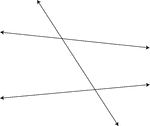### Transversal, 2 Lines Intersected By A

2 lines that are intersected by a third line known as a transversal.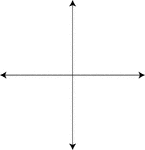### Intersecting Lines, 2 Perpendicular

2 intersecting lines, one of which is vertical and one of which is horizontal. The intersection forms…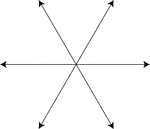### Intersecting Lines, 3

3 lines which intersect at a common point.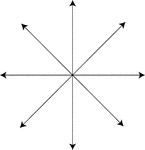### Intersecting Lines, 4

4 lines which intersect at a common point.### Intersecting Lines, 4

4 lines which intersect at a common point.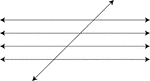### Transversal, 4 Parallel Lines Cut By A

4 parallel lines cut by a 5th line known as the transversal.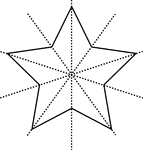### Star, 5-Point

Star with 5 points with lines of symmetry.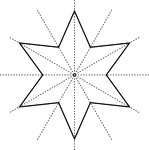### Star, 6-Point

Star with 6 points with lines of symmetry.### Intersecting Lines, 8

8 lines which intersect at a common point.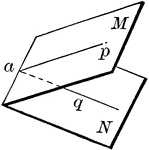### Dihedral Angle

A dihedral angle is the opening between two intersecting planes.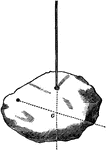### Finding the Center of Mass

"Let any irregularly shpaed body, as a stone or chair, be suspended so as to move freely. Drop a plumb…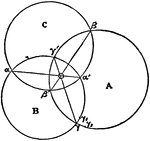### Circle Polar Point

Intersection of lines between a circle and its polar point.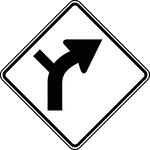### Combination Horizontal Alignment/Intersection, Black and White

"The horizontal alignment Turn signs may be used in advance of situations where the horizontal roadway…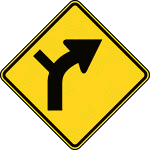### Combination Horizontal Alignment/Intersection, Color

"The horizontal alignment Turn signs may be used in advance of situations where the horizontal roadway…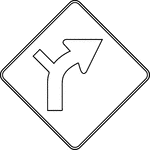### Combination Horizontal Alignment/Intersection, Outline

"The horizontal alignment Turn signs may be used in advance of situations where the horizontal roadway…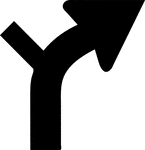### Combination Horizontal Alignment/Intersection, Silhouette

"The horizontal alignment Turn signs may be used in advance of situations where the horizontal roadway…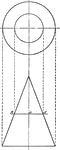### Intersection of a Cone and a Plane

Illustration of the intersection of a cone and a plane.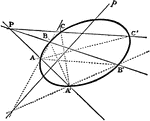### Conic Involution

The lines which join corresponding points in an involution on a conic all pass through a fixed point;…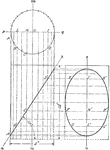### Plane Intersecting A Cylinder

Illustration of a cylinder cut by a plane making an angle of 57° with the base.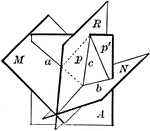### Plane Bisecting Dihedral Angle

Diagram used to prove the theorem: "Every point in a plane which bisects a dihedral angle is equidistant…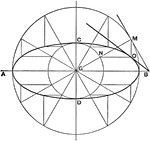### Ellipse Third Method

Draftsman's third method for drawing an ellipse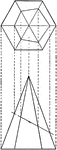### Intersection of Hexagonal Pyramid and a Plane

Illustration of the intersection of a hexagonal pyramid and a plane.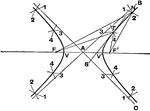### Hyperbola First Method

Draftsman's first method to draw a parabola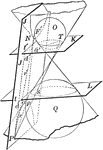### Cone Intersected by a Plane to Form a Hyperbola

Diagram depicting a cone with both nappes intersected by plane J to form a hyperbola.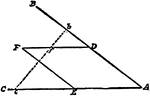### Pantograph

An instrument for making a reduced, enlarged, or exact copy of a plane figure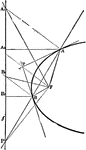### Parabola Foci Properties

The segments between the point of intersection of two tangents to a conic and their points of contact…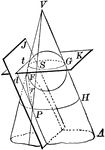### Cone Intersected by a Plane to Form a Parabola

Diagram of a cone intersected by plane J to form a parabola. Also pictured is a circle formed by the…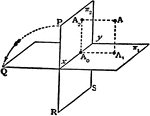### Perpendicular Planes

Two planes lie perpendicular to one another. A line perpendicular to plane 1 and a line perpendicular…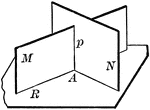### 2 Intersecting Planes Perpendicular To A Third Plane

"If two intersecting planes are each perpendicular to a third plane, their intersection is also perpendicular…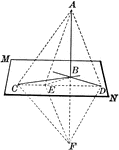### Line Perpendicular to Plane

If a straight line is perpendicular to each of two other straight lines in a plane at their point of…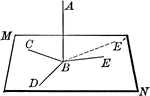### Line Perpendicular to Plane

Straight line AB is perpendicular to the lines in plane MN at point B.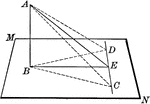### Line Perpendicular to Plane

Straight line AB is perpendicular to the lines in plane MN at point B. "If from the foot of a perpendicular…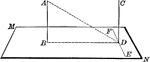### Lines Perpendicular to Plane

Two straight lines, AB and CD, perpendicular to the same plane (MN) are parallel.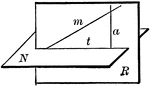### Plane Passed Perpendicular To A Given Plane

"Through a given line oblique to a plane, one, and only one plane, can be passed perpendicular to the…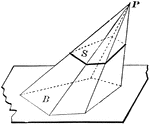### Pyramid Cut By Plane

Diagram used to prove the theorem: "If a pyramid is cut by a plane parallel to the base, the edges are…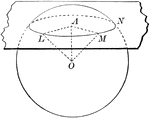### Sphere Cut By Plane

Diagram used to prove the theorem: "Every section of a sphere by a lane is a circle."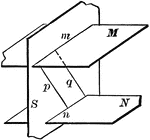### 3 Intersecting Planes

Illustration of three intersecting planes. "The intersections of two parallel planes by a third plane…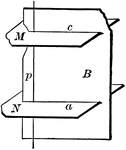### 3 Intersecting Planes

Illustration of three intersecting planes. "The straight line perpendicular to one of two parallel lanes…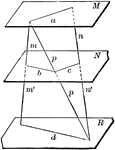### 3 Parallel Planes

"If two straight lines are cut by three parallel planes, the corresponding segments are proportional."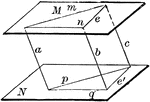### Angles In Parallel Planes

"If two angles not in the same plane have their sides respectively parallel and lying in the same direction,…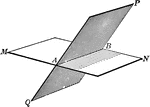### Intersecting Planes

The intersection of two planes is a straight line.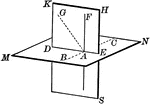### Intersecting Planes

The intersection of two planes is a straight line.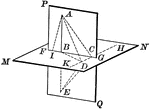### Intersecting Planes

The intersection of two planes is a straight line.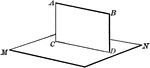### Intersecting Planes

The intersection of two planes is a straight line.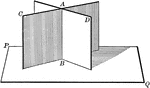### Intersecting Planes

Two intersecting planes on a third plane. "If two intersecting planes are each perpendicular to a third…### Intersecting Planes

Illustration of two intersecting planes.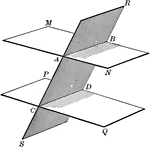### Parallel Planes

Two parallel planes cut by a third plane.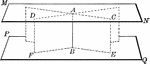### Parallel Planes

Two parallel planes perpendicular to a line (AB). "A straight line perpendicular to one of two parallel…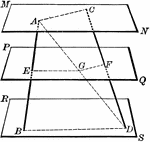### Parallel Planes Cut By 2 Lines

Three parallel planes cut by two lines.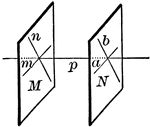### Parallel Planes

"If two intersecting lines are each parallel to a given plane, the plane of these lines is parallel…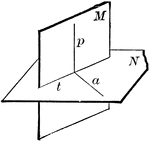### Perpendicular Planes

"If two planes are perpendicular to each other, a line in one of them perpendicular to the intersection…